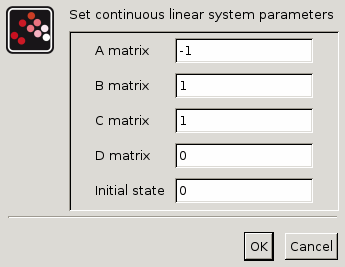Scilab Home page | Wiki | Bug tracker | Forge | Mailing list archives | ATOMS | File exchange
Change language to: Français - Português - 日本語 - Русский

See the recommended documentation of this function

# CLSS

Continuous state-space system

### Block Screenshot### Description

This block realizes a continuous-time linear state-space system.where x is the vector of state variables, u is the vector of input functions and y is the vector of output variables.

The system is defined by the (A, B, C, D) matrices and the initial state X0. The dimensions must be compatible.

### Dialog box• A matrix

A square matrix.

Properties : Type 'mat' of size [-1,-1].

• B matrix

The B matrix, [] if system has no input.

Properties : Type 'mat' of size ["size(%1,2)","-1"].

• C matrix

The C matrix , [] if system has no output.

Properties : Type 'mat' of size ["-1","size(%1,2)"].

• D matrix

The D matrix, [] if system has no D term.

Properties : Type 'mat' of size [-1,-1].

• Initial state

A vector/scalar initial state of the system.

Properties : Type 'vec' of size "size(%1,2)".

### Default properties

• always active: yes

• direct-feedthrough: no

• zero-crossing: no

• mode: no

• regular inputs:

- port 1 : size [1,1] / type 1

• regular outputs:

- port 1 : size [1,1] / type 1

• number/sizes of activation inputs: 0

• number/sizes of activation outputs: 0

• continuous-time state: yes

• discrete-time state: no

• object discrete-time state: no

• name of computational function: csslti4

### Example

This sample example illustrates how to use CLSS block to simulate and display the output waveform y(t)=Vc(t) of the RLC circuit shown below.The equations for an RLC circuit are the following. They result from Kirchhoff's voltage law and Newton's law.The R, L and C are the system's resistance, inductance and capacitor.

We define the capacitor voltage `Vc` and the inductance current `iL` as the state variables `X1` and `X2.`thusRearranging these equations we get:These equations can be put into matrix form as follows,The required output equation isThe following diagram shows these equations modeled in Xcos where R=10Ω, L=5 mΗ and C=0.1µF; the initial states are x1=0 and x2=0.5.

To obtain the output Vc(t) we use CLSS block from Continuous time systems Palette.### Interfacing function

• SCI/modules/scicos_blocks/macros/Linear/CLSS.sci

### Computational function

• SCI/modules/scicos_blocks/src/c/csslti4.c (Type 4)